# Scientific and Engineering Notation Scientific Engineering Notation This

• Slides: 15Scientific and Engineering Notation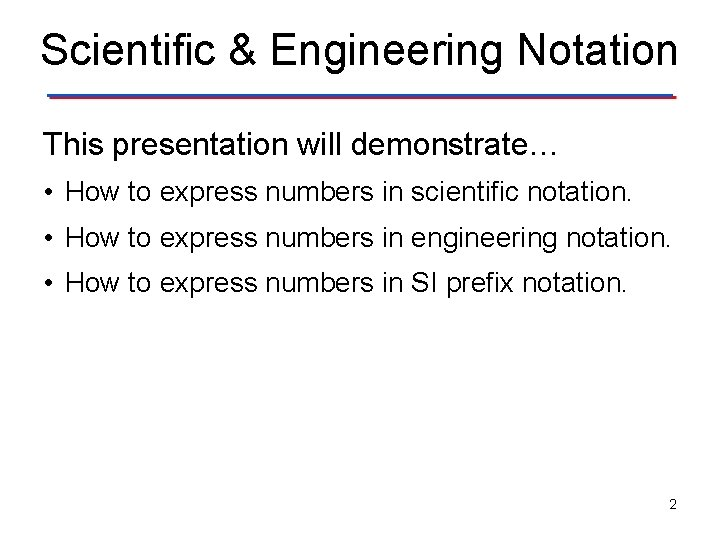Scientific & Engineering Notation This presentation will demonstrate… • How to express numbers in scientific notation. • How to express numbers in engineering notation. • How to express numbers in SI prefix notation. 2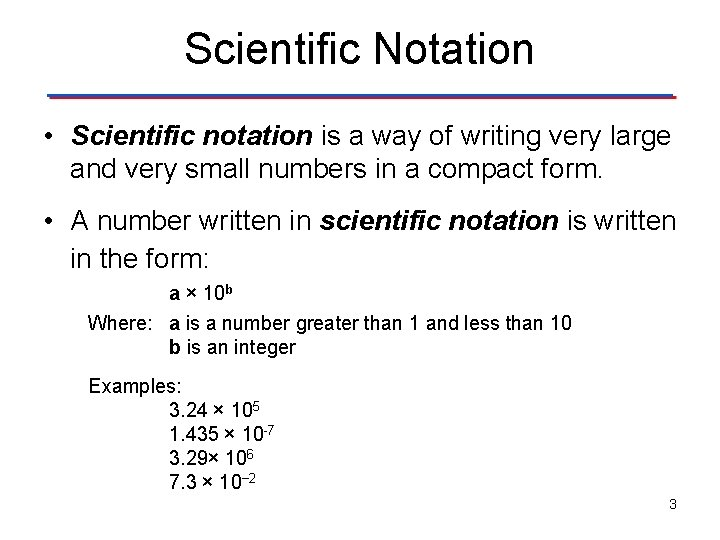Scientific Notation • Scientific notation is a way of writing very large and very small numbers in a compact form. • A number written in scientific notation is written in the form: a × 10 b Where: a is a number greater than 1 and less than 10 b is an integer Examples: 3. 24 × 105 1. 435 × 10 -7 3. 29× 106 7. 3 × 10− 2 3Writing A Number in Scientific Notation • Shift the decimal point so that there is one digit (which cannot be zero) before the decimal point. • Multiply by a power of 10, equal to the number of places the decimal point has been moved. • The power of 10 is positive if the decimal point is moved to the left and negative if the decimal point is moved to the right. 4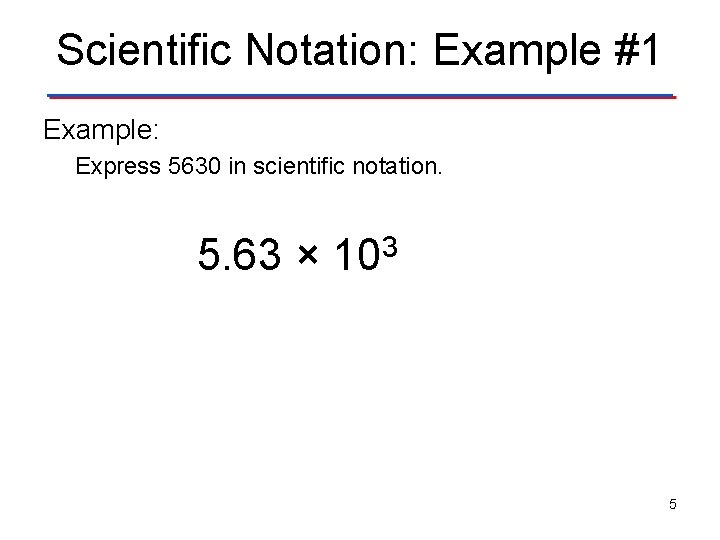Scientific Notation: Example #1 Example: Express 5630 in scientific notation. 5. 63 × 103 5Scientific Notation: Example #2 Example: Express 0. 000628 in scientific notation. 6. 28 × 10− 4 6Engineering Notation • Engineering notation is similar to scientific notation. In engineering notation the powers of ten are always multiples of 3. • A number written in engineering notation is written in the form: a × 10 b Where: a is a number greater than 0 and less than 999 b is an integer multiple of three Examples: 71. 24 × 103 4. 32 × 10 -6 320. 49 × 109 123. 452 × 10− 12 7Writing A Number in Engineering Notation • Shift the decimal point in “groups of three” until the number before the decimal point is between 0 and 999. • Multiply by a power of 10 that is equal to the number of places the decimal point has been moved. • The power of 10 is positive if the decimal point is moved to the left and negative if the decimal point is moved to the right. 8Engineering Notation: Example #1 Example: Express 16346000000 in engineering notation. 16. 346 × 109 9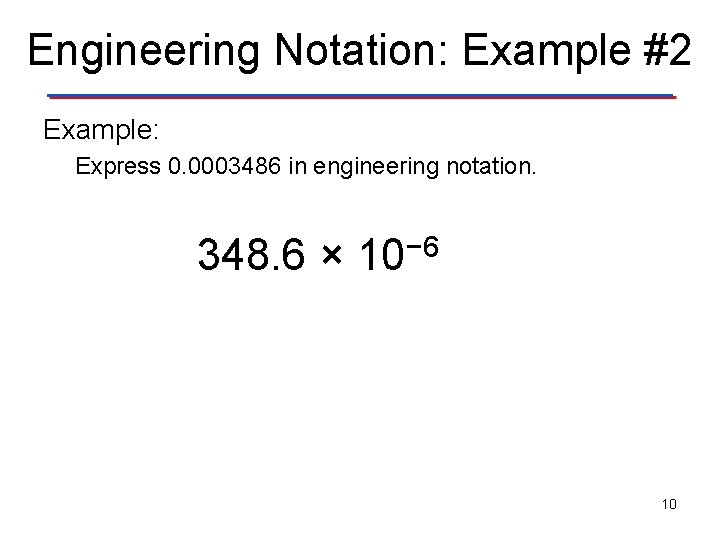Engineering Notation: Example #2 Example: Express 0. 0003486 in engineering notation. 348. 6 × 10− 6 10SI Prefixes • SI prefixes are a shorthand way of writing engineering notation for SI numbers. • The International System of Units (abbreviated SI from the French Système International d'Unités) is the modern form of the metric system. It is the world's most widely used system of units for science and engineering. 11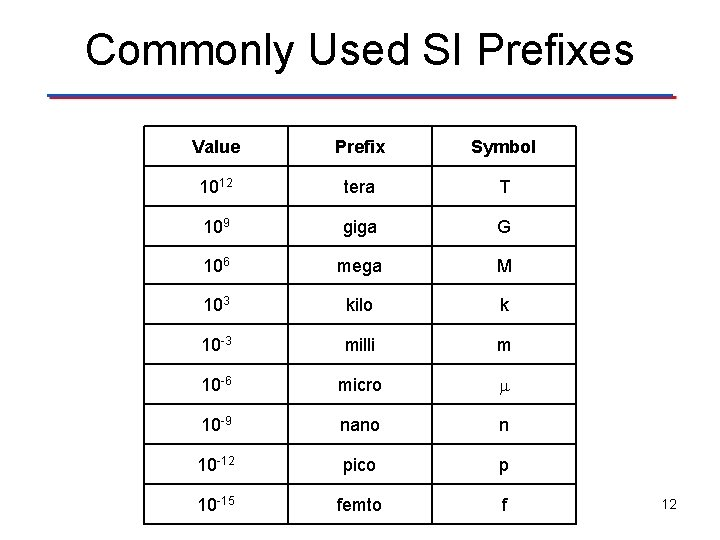Commonly Used SI Prefixes Value Prefix Symbol 1012 tera T 109 giga G 106 mega M 103 kilo k 10 -3 milli m 10 -6 micro 10 -9 nano n 10 -12 pico p 10 -15 femto f 12SI Notation: Example #1 Example: Express 27500 using standard SI notation. (Note: is the Greek letter omega. In electronics, it is the symbol used for resistance. ) 27. 5 × 103 27. 5 k 13SI Notation: Example #2 Example: Express 0. 000568 Volts using standard SI notation. 568 × 10 -6 568 μV 14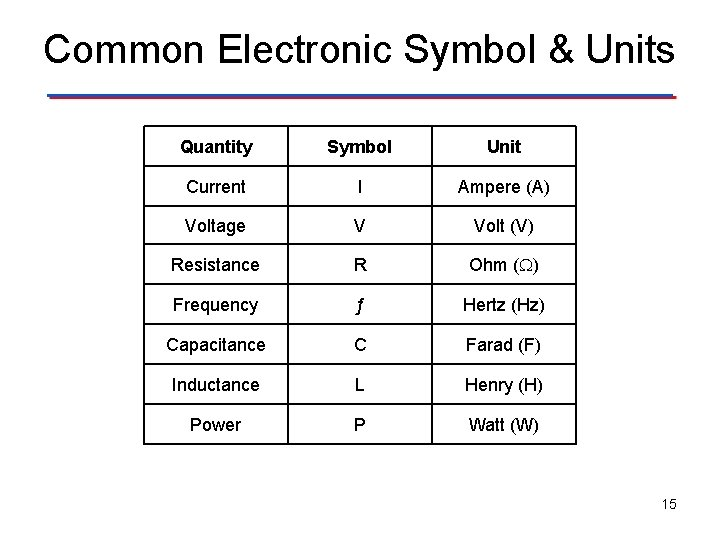Common Electronic Symbol & Units Quantity Symbol Unit Current I Ampere (A) Voltage V Volt (V) Resistance R Ohm ( ) Frequency ƒ Hertz (Hz) Capacitance C Farad (F) Inductance L Henry (H) Power P Watt (W) 15